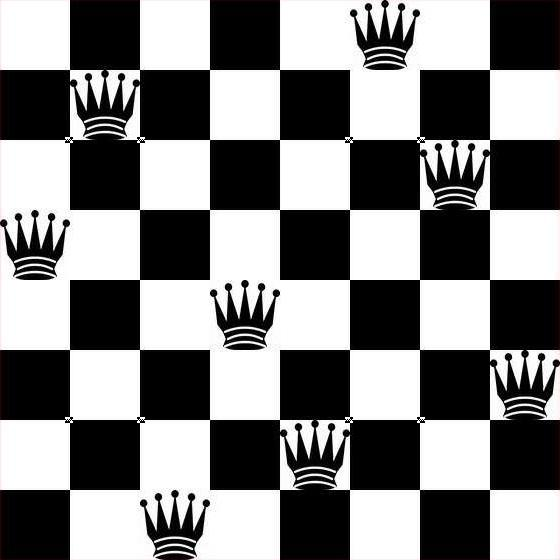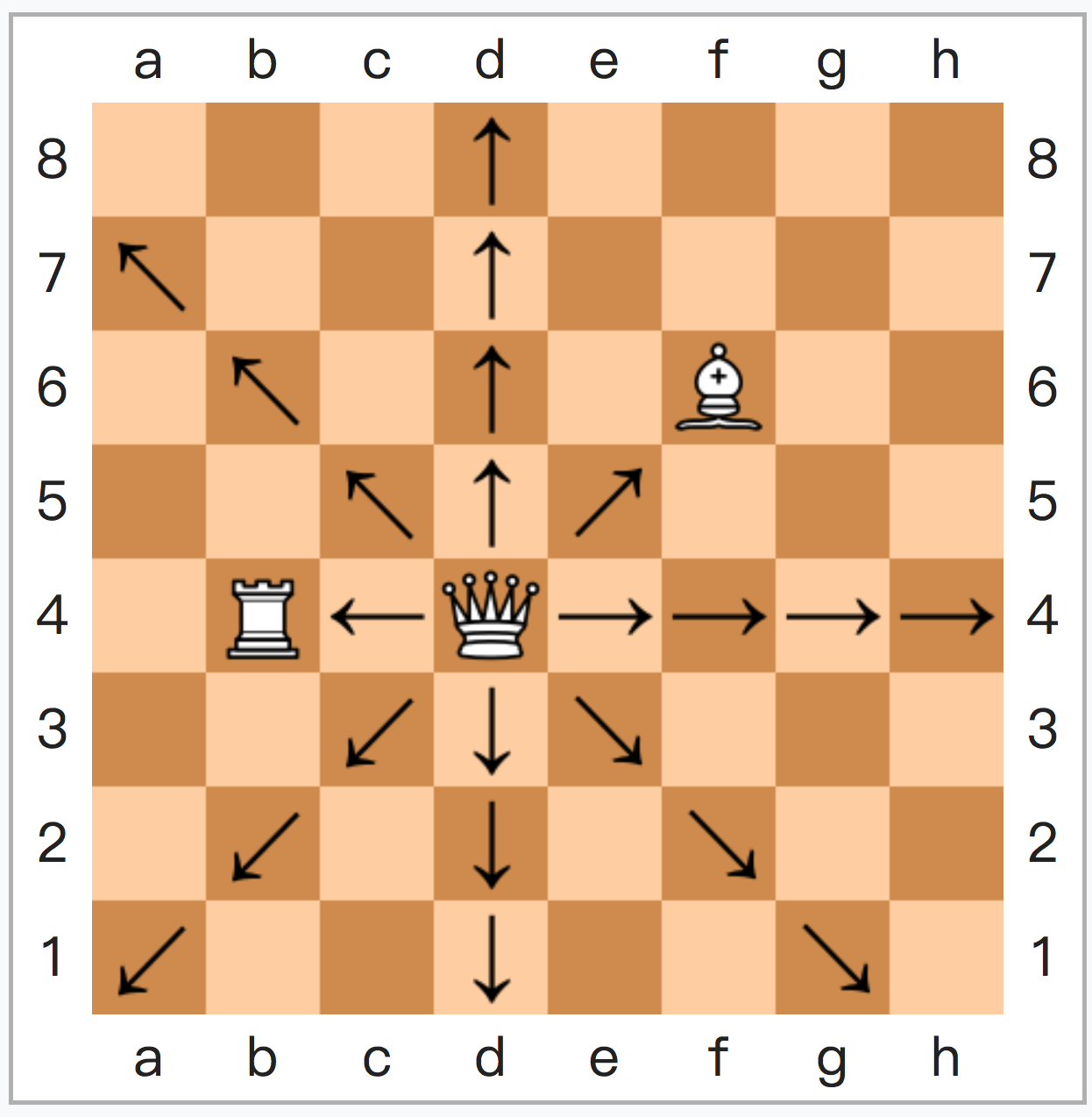## 【为什么系列】为什么我读不懂数学书？

### ——那是因为我搞不清形式语言和非形式语言 (v0.02)

1 将非形式化的理论“形式化”，得到
2 （一般的）形式体系和特殊的形式体系——对象理论，而后
3 借用元理论，描述并研究形式体系 最后使你到到达了数学的世界

## The Way to an ‘Impossible Program’ 1: Automaton, Algorithm, and Church-Turing Thesis

Outputs from Computation Theory Lectures

From the Automata Theory before we moved to Computability Theory and Computational Complexity Theory, we had gone through several Formal Languages, Formal Grammars and Abstract Machines. For the lecture of COS487/MAT407 in Princeton, Formal System was the next topic for students’ discussion; but as long as I’m writing these posts in a line for everyone has the same amateur knowledge basis like me, I’d like to put the First-Order-Logic a bit off, and continue to explaining the Computability Theory in advance.

Speaking of those Abstract Machines, we can see the limitations each one of them easily: Due to the absence of storage, a Finite State Machine (FSM) can’t deal with those problems with ‘counting infinitely’ (eg., determine if a string ‘balanced’ with a and b, or $$L = \{a^n b^n | n ≥ 0 \}$$); a Pushdown Automaton (PDA) has infinite storage, but that storage is still a stack. That means a PDA must consume the input characters ‘in the order’ in which they are received, and cannot access them again, except by placing them on the stack. Therefore, a PDA can’t determine if a string satisfies such rules like ‘a string has the same amount of particular symbols in total’ (eg. “aacbcbdd”); besides, it only works on Context-Free…

Until we got A Turing machine – it has unlimited storage can be visited so efficiently under any order, any loop, any condition and any sub-function. Life is great ;D

Until, we reached the boundary of it. We added more features to those Turing machines but didn’t expand any additional abilities to them, by proving that those features can be replaced by another (bunch of) designed Turing Machines, if those features are applicable. Then where is that limitation of a Turing Machine? Is there an impossible program?

Those ‘General Systems’ such as Turing Machines are dealing with algorithms, not to mention computers. Then, to answer those questions, we have to see the features of an Algorithm. If we know the algorithms’ features and abilities, we might figure out what those limitations are.

In a total rudimentary way to explain, an algorithm is a set of instructions, each instruction takes an/ε input(s) and got an output of it/them. But there are 4 rules applied:

• The number of instructions are finite;
• The instructions can’t be complex;
• The whole process with instructions can be done in finite length of time, if those instructions are followed/used correctly;
• The result out of those sets of instructions must be ‘correct’ if those instructions are followed/used correctly.

Based on the 4 rules above, we can turn numerous algorithms into programming codes. But is it possible to convert each and every algorithm into a set of instructions for general systems’ execution? People (especially people writing codes) may just answer ‘yes’, following their instincts. Yet under the realm of Computational Architecture, the direct, abstractive idea of an algorithm is totally unequal to its logical, concrete implementation.

So again, can we design an algorithm with such unbelievable complexity and unmeasurable scale that no machine can grasp its stem?

Since 1930s, computer scientists have been working on creating an algorithm which could not be ‘solved’ by an abstract machine – every attempt of creating an model of computation returns a system, and those systems were all proved to have the equivalent capacity of a Turing machine, whereas there’s still no mathematical / logical proof for that general question. In fact, from the place I put up the 4 rules for algorithm, we are not be able to talk about the whole question on the level of logic or mathematics any more. But at least, along the way of exploration, we are confident to accept the hypothesis below:

“Every algorithm has the features as I mentioned in this post, can be executed by an abstract machine, or specifically, a Turing machine.” or let’s say, “a function on the natural numbers can be calculated by an effective method if and only if it is computable by a Turing machine.”

And here we reached the first stop of the Computability Theory: Church-Turing Thesis,

“We shall use the expression ‘computable function’ to mean a function calculable by a machine, and let ‘effectively calculable’ refer to the intuitive idea without particular identification with any one of these definitions.”

Turing, Alan (1938). Systems of Logic based on Ordinals (PhD thesis). Princeton University. doi: 10.1112/plms/s2-45.1.161.

or in Gandy’s(1980:123) words, every effectively calculable function is a computable function.

Despite of the form of Church-Turing Thesis is a ‘thesis’, a literature statement than a logical, formal expression, the Church-Turing Thesis came out from solid formalisms: the λ-calculus, besides recursion, and the Turing machine. Those are the topics up next, which requires we write something to show the power of those 3.

We’ll begin from the λ-calculus.

## 【公式编辑测试+小题目w】1 试计算概率论公式：

$\varphi(x)=\frac{1}{2\pi\sigma_1\sigma_2}\int^{+\infty}_{-\infty}e^{-\frac{\xi^2}{2\sigma_1^2}}e^{-\frac{(x-\xi)^2}{2\sigma_2^2}}d\xi\quad(\delta_1>0,\delta_2>0).$

2 契贝谢夫-厄尔米特多项式由公式
\begin{equation}
\end{equation}

\begin{equation}
\int^{+\infty}_{-\infty}H_m(x)H_n(x)e^{-x^2}dx=
\left\{
\begin{aligned}
&0,& 若m\neq n\\
&2^nn!\sqrt{\pi},&若m=n
\end{aligned}
\right.
\end{equation}

3 用latex编公式好好玩www

$\forall_{x_i}\exists_{a}a \wedge b \vee \neg c$什么的www

3839的答案为$(\varphi)x=\frac{1}{\sigma\sqrt{2\pi}}e^{-(\frac{x^2}{2\sigma^2})},$

## N个女人的老死不相往来——n皇后问题n皇后问题，较早为八皇后问题，出现于1848年。
Max Bezzel讨论的是“在国际象棋上出现8个后时，（八皇后问题的其中一个解）

（一方全升变，一方被吃过路兵一次，加上初始两个）

——直到图论和函数式编程思想出现，

' n queens cast by go basic
[loop]
input "How many queens (N>=4)";n
if n < 4 then
print "Must be greater than 4"
goto [loop]
end if

dim plot$(100,100) dim q(n+20) dim e(n+20) dim o(n+20) r=n mod 6 if r<>2 and r<>3 then gosub [samp] goto [shoBoard] end if for i=1 to int(n/2) e(i) = 2 * i next for i=1 to int((n/2)+.5) o(i) = 2 *i-1 next if r = 2 then gosub [edt2] if r = 3 then gosub [edt3] s = 1 for i=1 to n if e(i)>0 then q(s) = e(i) s = s+1 end if next for i=1 to n if o(i) > 0 then q(s) = o(i) s = s + 1 end if next ' print board [shoBoard] cls for i = 1 to n plot$(i,26-q(i)) = "*"
plot$(i,24-n) = chr$(96+i)
plot$(n+1,26-i) = str$(i)
next i
for ii = 1 to 100
for jj = 1 to 100
print left$(plot$(jj,ii)+" ",1);
next jj
print
next ii
end

' the simple case
[samp]
p = 1
for i = 1 to n
if i mod 2=0 then
q(p) = i
p    = p + 1
end if
next i
for i = 1 to n
if i mod 2 then
q(p) = i
p    = p + 1
end if
next
return
' edit list when remainder is 2
[edt2]
for i=1 to n
if o(i) = 3 then
o(i) = 1
else
if o(i)=1 then o(i) = 3
end if
if o(i) = 5 then
o(i)= o(i) -1
else
if o(i) = 0 then
o(i) = 5
return
end if
end if
next

' edit list when remainder is 3
[edt3]
for i = 1 to n
if e(i) = 2 then
e(i)  = e(i)-1
else
if e(i) = 0 then
e(i) = 2
goto [more]
end if
end if
next i
' edit list some more
[more]
for i = 1 to n
if (o(i)=1 or o(i)=3) then
o(i) = o(i)-1
else
if o(i) = 0 then
o(i)   = 1
o(i+1) = 3
return
end if
end if
next


# Brute force permutations for 8 queens by R

safe <- function(p) {
n <- length(p)
for (i in seq(1, n - 1)) {
for (j in seq(i + 1, n)) {
if (abs(p[j] - p[i]) == abs(j - i)) return(F)
}
}
return(T)
}

queens <- function(n) {
p <- 1:n
k <- 0
while (!is.null(p)) {
if(safe(p)) {
cat(p, "\n")
k <- k + 1
}
p <- next.perm(p)
}
return(k)
}

queens(8)


——只会利用工具的人，终究会被工具抛弃。

N皇后问题中，穷举法的复杂度为(n!)，1 N皇后于NxN棋盘上，若老死不相往来，则绝不在同一行列。
2 攻击范围有重叠时，绝非边与边重叠，只可相交，且相交仅会出现一次。

（计算第5横行时，下子状态保留为 0001 0000，

#include <iostream>

// total:记录解；finishline:确认的行
int total = 0, finishline = 1;

// row:竖直攻击; ls:斜杠攻击; rs:反斜杠攻击
void trying(long row, long ls, long rs)
{
if (row != finishline) // 开始新行
{
long position = finishline & ~(row | ls | rs);
while(position) // 若行间无位可放...
{
long pointline = position & -position;
position -= pointline;
trying(row + pointline, (ls + pointline) << 1, (rs + pointline) >> 1);
}
}
else
{
total++;
}
}

using namespace std;

int main(int argc, char *argv[]) {
int grid = 8; // 修改grid的话，1～31皇后都能解w

finishline = (finishline << grid) - 1;

trying(0, 0, 0);

cout << total << endl;

return 0;

}


#include <iostream>
int total = 0, finishline = 1;
void trying(long row, long ls, long rs){
if (row != finishline) { long position = finishline & ~(row | ls | rs);
while(position) { long pointline = position & -position; position -= pointline; trying(row + pointline, (ls + pointline) << 1, (rs + pointline) >> 1); }} else {total++;}}
using namespace std;
int main(int argc, char *argv[]) {
int grid = 8; // 修改grid的话，1～31皇后都能解w
finishline = (finishline << grid) - 1; trying(0, 0, 0); cout << total << endl;
return 0;}


// 此处需使用八皇后算法，但忽略计算过程，仅使用答案节省时间。
// 八皇后解枚举数据头在******
void 8queensAnswers (0, 0, 0, 0)
//在里面做一个调用92个答案的接口，传参出去给大结构体
//保存各个答案类似于[91, 0, 4, 7, 5, 2, 6, 1, 3]这样
//或者n皇后的话[0,1][1,0][2,0][3,2][4,10][5,40]...这样


N皇后问题——记录下那些老死不相往来的N个女人的位置吧

## (Syntax Highlight Testing#include &lt;iostream&gt;

using namespace std;

//此处开始搞事情

int main(int argc, char *argv[]) {

//此处开始搞事情

cout &lt;&lt; "此处开始练傻" &lt;&lt; endl;
return 0;
}